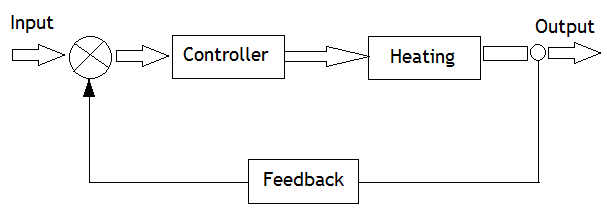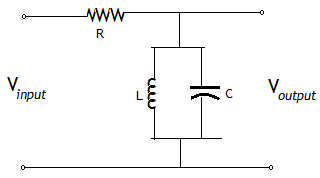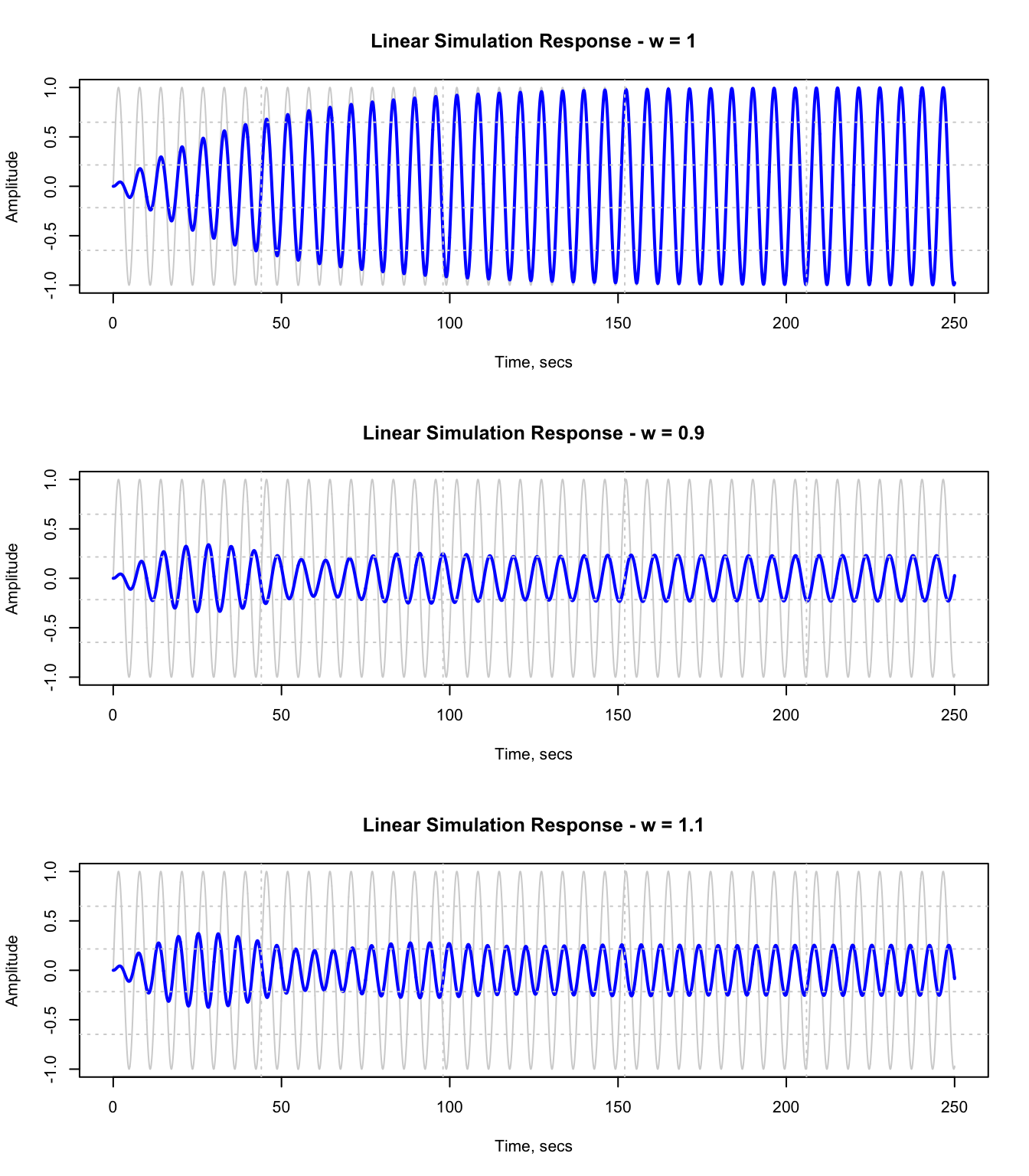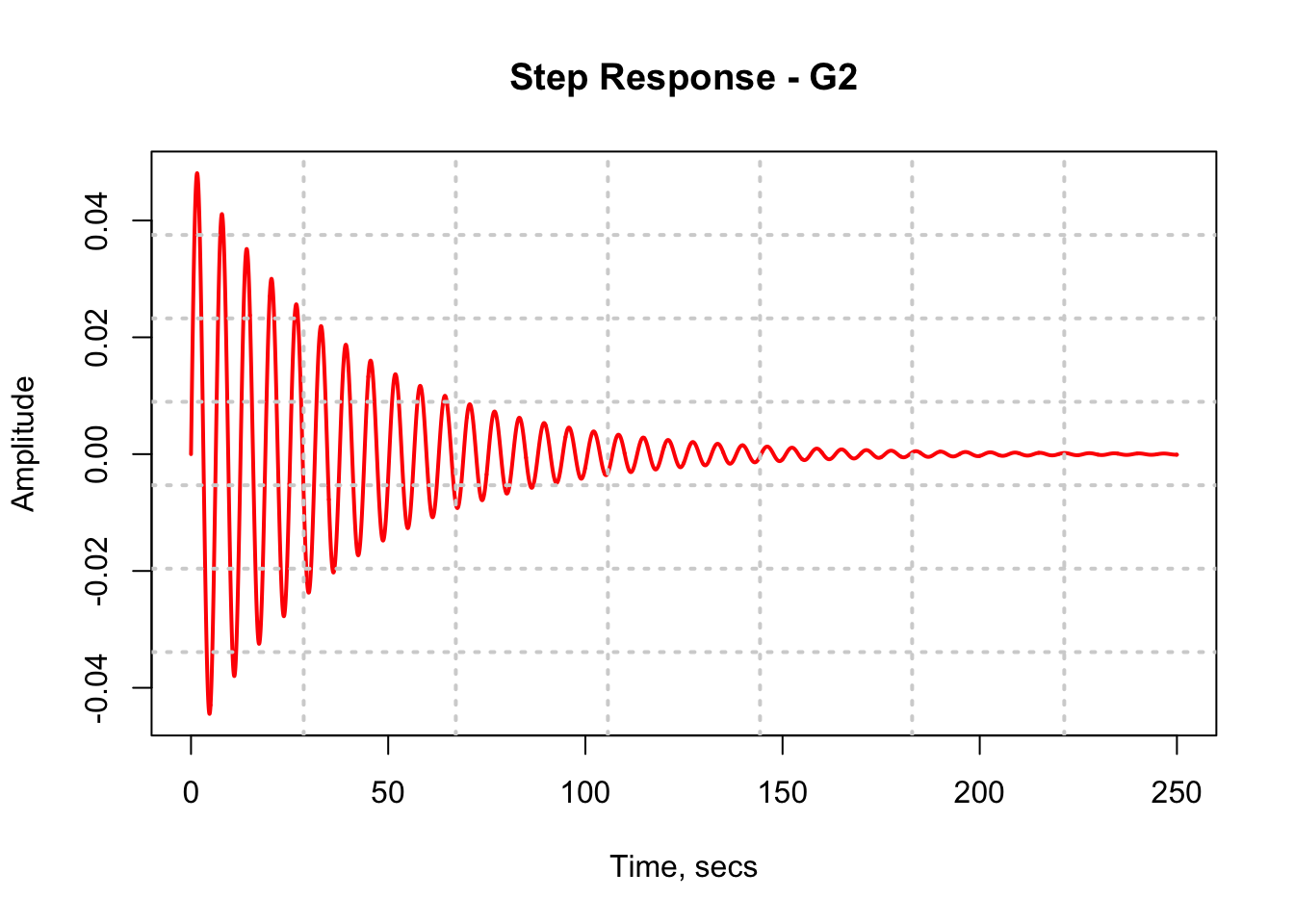# Control Systems Toolbox in R - a GSoC 2017 Project

by Ben Ubah

## Introduction

Control theory is an interdisciplinary branch of mathematics and engineering that has the objective of controlling physical systems. A control system is a device or a collection of devices that manage, command, direct or regulate the behavior of other devices or systems. Control systems engineering is a major application of control theory, and involves the study, design/modeling of automatic control systems. Control theory has also been applied to several other fields such as finance, sociology, psychology, physiology and other sciences that analyze dynamic systems.

In recent times, control systems have played a major role in the development and advancement of modern technology and civilization. Control systems are essential in any field of engineering and science, as they are an important and integral part of systems such as space-vehicle systems, robotic systems, modern manufacturing systems, nuclear reactors, navigation systems and industrial operations involving control of temperature, pressure, humidity, flow, speed/velocity, etc.

### Example of a Control System

Imagine the operation of an Automatic Electric Iron where its heating elements are controlled by the temperature output of the iron. As long as there is power supply to the electric iron, and a desired heat-level is selected, the electric iron automatically controls at what time to turn-on and switch-off heating so as to maintain that temperature. This type of control system is called a feedback control system: a system in which the output is fed back to the input as a control signal.

Figure 1 shows a simple block diagram depicting a sample feedback control system for a heating system.Figure 1: Block Diagram Model for a Heating System

Another example is the control system for an aircraft or a space shuttle. Truly, such control system would be complex as there are several sub-systems that are interconnected in such transport systems. Therefore, it is very necessary to simulate how these systems could behave using a computer before implementing them physically. This is the reason why computer simulation of control systems have been proliferated.

### Control Systems R-GSoC Project

For this Google Summer of Code (GSoC) 2017 project, we are working to develop a control system toolbox for the R language. The rationale is that there is a control toolbox for most commercial and open-source technical computing platforms out there, and while the R language is experiencing tremendous growth in its capabilities and user base, it seems necessary to extend the language to serve other scientific and engineering communities. For example, in Python, the development of NumPy and the general SciPy stack, added some dimensions and attracted several scientific domains to the use of Python. This GSoC project hopes to make basic design, analysis and simulation of control systems possible within the R ecosystem.

The major objective of this year’s GSoC project is to try to:

• Design and develop a framework for the package that defines data structures and creates flexibility of usage and extension for future users and interested contributors to easily adopt the package. The framework is also expected to mimic the I/O style of existing control systems toolkits.
• To port a set of functions from the RLabPlus control-toolbox and adapt them to the defined data structures and general framework.
• To test the functions, create documentation, usage examples and build them for distribution.

### Benefits of a Control Systems Toolbox in R

Some benefits of having a control-systems-toolbox in R are:

• A control package in R could be useful to engineers and students who are looking to migrate to an open-source language like R that has better visualization options and interfaces to several technologies. Models for several dynamic systems could then be analyzed using R.
• The package could assist in further developments in areas like optimal control theory which has several applications to economics and management sciences.

### Current State of the Project

At the time of writing this article, the first phase of coding for GSoC has elapsed, and we are delighted to meet our target of creating 18 functions that define the general framework and structures. Consequently, a user can, at this stage, perform the following:

• Create LTI system models of state-space, transfer function and zero-pole classes
• Conversions from one system model to other system models
• Convert continuous-time system models to discrete-time models
• Obtain time response for LTI systems
• Install the development version of the package using: devtools::install_github(“benubah/control”)

### Practical Example

#### Analyzing the Time Response of a Bandpass RLC Filter Circuit

Wikipedia: “A bandpass filter is a device that passes frequencies within a certain range and rejects (attenuates) frequencies outside that range.” Bandpass filters are commonly used in wireless transmitters and receivers to prevent transmitters from interfering with other stations and to allow signals within selected frequency ranges to be decoded, while preventing signals at unwanted frequencies from passing through to the receiver. In astronomy, bandpass filters are used to allow only certain portion of light spectrum pass through to an instrument. They have several other applications in neuroscience, digital signal processing, atmospheric sciences, etc.

The following example is taken from a Mathworks web-page and it shows how to analyze the time response of a common RLC bandpass filter circuit using the current available functions of the Control Systems Toolbox in R. The attenuation properties of the filter circuit, G2 can be ascertained by simulating how this filter transforms sine waves with frequency 0.9, 1, and 1.1 rad/s.Figure 2: Bandpass RLC Filter Circuit.

For this example, we will try to show how the following could be achieved using the developing control library:

• Creating a transfer function model
• Converting a transfer function to state-space representation
• Converting a continuous-time model to discrete-time model
• Performing Linear simulation using lsim
• Obtaining step response using lsim

The following values are given R = 1, L = 1, C = 1, R2 = 20

The transfer function for this circuit is:

$G(s) = \frac{V_output}{V_input} = \frac{s/(RC)}{s^2 + s/(RC) + 1/(LC)}$

On the R Console (or in an R script), after installing the control package, the following code obtains the time response of the circuit:

library(control)
##
## Attaching package: 'control'
## The following object is masked from 'package:base':
##
##     append
R <- C <- L <- 1
R2  <-  20
t  <-  seq(0,250,0.05)
G1 <-  tf(c(1/(R*C), 0), c(1, 1/(R*C), 1/(L*C) ) )
G2  <-  tf(c(1/(R2*C), 0), c(1, 1/(R2*C), 1/(L*C) ) )
print(G1)
##
## y1:
##              s
##   - - - - - - - - -
##      s^2 +  s + 1
##
##
## Transfer Function: Continuous time model
tf2ss(G1) # example: convert the transfer function to state-space representation
##
##  sys.A =
##
##      x1   x2
## x1   -1   -1
## x2    1    0
##
## sys.B =
##
##      u1
## x1    1
## x2    0
##
## sys.C =
##
##      x1   x2
## y1    1    0
##
## sys.D =
##
##      u1
## y1    0
##
##
##  State-Space system: Continuous time model
c2d(G1, 0.1) # example: convert the system G1 from continuous-time to discrete-time model
##
## y1:
##   0.09500408 z^1 - 0.09500408
##   - - - - - - - - - - - - - - - - -
##      z^2 - 1.895329 z + 0.9048374
##
##
## Sample Time = 0.1
## Transfer function: Discrete time model
response <- lsim(G2,sin(t),t)
par(mfrow = c(3,1))
plot(t, sin(t), type = "l", xlab = "Time, secs", ylab = "Amplitude", main = "Linear Simulation Response - w = 1", col = "lightgray")
lines(t, response$y, type = "l", col = "blue", lwd = 2) grid(5,5, col = "lightgray") response2 <- lsim(G2, sin(0.9*t), t) plot(t, sin(t), type = "l", xlab = "Time, secs", ylab = "Amplitude", main = "Linear Simulation Response - w = 0.9", col = "lightgray") lines(t, response2$y, type = "l", col = "blue", lwd = 2)
grid(5,5, col = "lightgray")

response3 <- lsim(G2, sin(1.1*t), t)
plot(t, sin(t), type = "l", xlab = "Time, secs", ylab = "Amplitude", main = "Linear Simulation Response - w = 1.1", col = "lightgray")
lines(t, response3$y, type = "l", col = "blue", lwd = 2) grid(5,5, col = "lightgray")Figure 1: Figure 3: R Plot for Linear Simulation Response of RLC bandpass circuit The output of the simulation displayed in Figure 3 shows a pure sine wave (in light-gray color) behind the wave response from our linear simulation (in blue). At w = 1 rad/s, after the transients have died off, the wave remains a pure sine wave – no attenuation observed. However, the waves at 0.9 and 1.1 rad/s are significantly attenuated. Please get the code here: https://github.com/benubah/controldev/blob/master/R/lsimExample.R To obtain a step response for the RLC filter circuit using lsim as shown in Figure 4, the following code is used: dims <- dim(as.matrix(t)) u <- matrix(rep(1,dims), dims, dims) stepresponse <- lsim(G2, u, t) plot(t, stepresponse$y, type = "l", xlab = "Time, secs", ylab = "Amplitude", main = "Step Response - G2", col = "red", lwd = 2)
grid(7,7, col = "lightgray", lwd = 2)Figure 2: Figure 4: R Plot for Step Response of the RLC bandpass circuit

### Next Coding Phase

In the next coding stage, we plan to attempt creating functions that would perform the following:

• Evaluate transfer function expressions in the s-domain within R as part of the framework.
• Control system block diagram algebra functions – system interconnections

### Challenges

The expected challenge for this project is to accurately set up a framework that defines data structures and how functions operate within the framework in such a manner that would be attractive (due to simplicity, reusability and extensibility) to prospective users and contributors.

To overcome this challenge, through the assistance of my mentors, we have examined the data architectures and plotting layout of other open-source scientific languages, and from these, designed a plan and framework that would integrate reasonably with the architecture of the R language while remaining open to improvements.

### Dependencies

The Control Systems Toolbox for the R language is expected to depend on R packages like, pracma, signal and expm.

### Feedback

We would be glad to hear from the R community – feel free to get in touch with any suggestions, opinions or comments.

### Conclusion

In this article, we have introduced the Control Systems Toolbox GSoC 2017 project, its current state, benefits and practical examples showing some functions that are currently available. We have also listed the future coding plans for the next GSoC coding phase. We hope to be able to update the R community as things develop.

Share Comments · · · ·